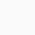Course

# Machine Dynamics with MATLAB

👤 Join WhatsApp Learners
New here?
Deal Score0

Are you keen to design a vehicle suspension using MATLAB? In this course, you will start at the very beginning with dynamic modeling, simulation, and analysis of a technical system using different MathWorks products.

### Join Email Learners

Follow the guide on landing page

This course will introduce you to a broad range of methodologies used in machine dynamics. You will learn how to model a vehicle using the fundamentals of mechanics. You will get a deep understanding of the equations of motion and how to solve them using powerful MathWorks tools. Eventually, you will gain the ability to analyze and interpret the computational results to optimize your design.

To get the most out of your time, the course is subdivided into five weeks: lectures, tutorials, and exercises. During lectures, you will get all the theoretical background of machine dynamics. Tutorials will teach you the basics of MathWorks products, and exercises will merge your theoretical knowledge with the practical use of the software into an exciting application.

You will learn how to model a vehicle by a one and a two-degree of freedom system. These systems could be base excited, force excited, not excited, damped, or undamped. Their mathematical representation could be solved analytically, by state-space representation or by solving the differential equation itself.

Therefore, if you have ever wondered how to design a vehicle suspension using MathWorks tools, we recommend attending this course.

• Basic and advanced MATLAB skills
• Define a sensible modeling depth
• Derive equations of motion for different models
• Solve the equations of motion using different approaches
• Presentation of results using animations

Week1: Basics of oscillation
Definition of important parameters of an oscillation. Introduction to the methods of
modeling and discussion about modeling depth. Derivation of an equation of motion of a
translational one degree of freedom system.

Week2: Discrete systems with one degree of freedom and their Eigen behavior
Analytical solution of an equation of motion and animation of the results. Analysis of
three typical cases of eigen behavior. Introduction to state-space representation and
numerical solution of an equation of motion. Getting started with Simulink.

Week3: Discrete systems with one degree of freedom with forced excitation
Description of different types of excitation. Derivation and solution of an equation of
motion with frequency-dependent force excitation and frequency independent force
excitation, respectively. Extension of the model to base excitation and explanation and
development of effective vibration insulation.

Week4: Discrete systems with multiple degrees of freedom and their Eigen behavior
Derivation of a system of equations of motion that describes vertical dynamics and
pitch motion. Analytical solution of this system and discussion of the homogeneous
solution. Analyzes three typical cases of motion.

Week5: Discrete systems with multiple degrees of freedom and their Eigen behavior
and with forced excitation
Transfer of equations into state-space representation and a Simulink model.
Application of frequency-independent and frequency-dependent force excitation to the
system of equations of motion. Adaption of the model to base excitation. Development of
a vibration absorber.

Week6: Discrete systems with multiple degrees of freedom with forced excitation
Adaption of the model to base

0DISCLAIMER: Courses on Future Syllabus are free but subject to return to their original prices on host platforms upon coupon expiration. Enrol while they are free.#### Silas Bamigbola

Certified Computer Engineer & Author, 'Lost Boys'. I believe in the influence of right information. Join me on Twitter.

Stay activeRegister New Account